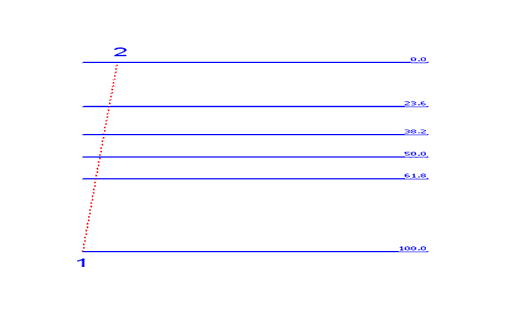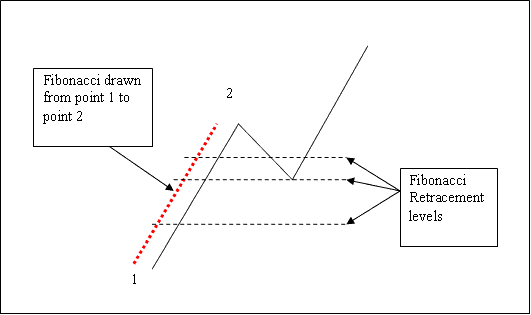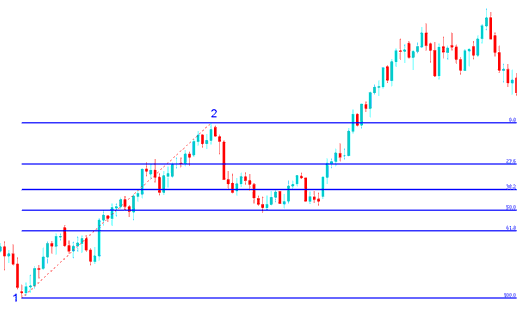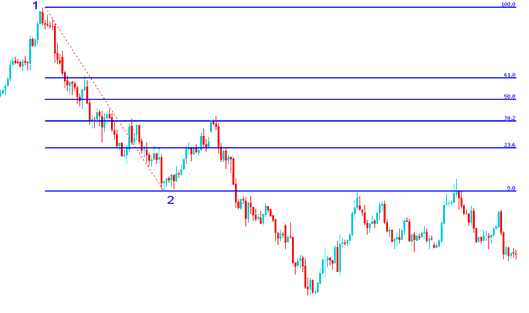# How to Draw Fibonacci Stock Indexes Indicator Tool on Stock Indexes Charts

## How Do I Use Indices Trading Fibonacci Retracement Indicator?

The indices trading Indices Trading Fib retracement levels are explained below: indices traders use this Indices Trading Fibonacci Retracement Levels stock indices indicator to determine where to open a trade.

Traders use Indices Trading Fib retracement tool to determine where to place a buy indices trade in a upward indices trend and where to place a sell indices trade in a downward indices trend.

## How to Draw Indices Trading Fib Retracement

To Draw the Indices Trading Fib retracement indicator the first thing to know is that this Indices Trading Fibonacci Retracement tool is always drawn in the direction of the indices trend.

If the indices trend is upwards the Indices Trading Fibonacci Retracement indicator is drawn upwards.

If the indices trend is downwards the Indices Trading Fibonacci Retracement indicator is drawn downwards.

How to Draw Indices Trading Fib Retracement - How to Draw Indices Trading Fibonacci RetracementHow to Draw Indices Trading Fib Retracement - How to Draw Indices Trading Fibonacci Retracement

How to Draw Fibonacci Stock Indexes Indicator Tool on Indices ChartsHow to Draw Fibonacci Stock Indexes Indicator Tool on Indices Charts

## How to Draw Indices Trading Fib Retracement in an Upward Indices Trend

In the technical analysis example explained and illustrated below of how to draw Indices Trading Fibonacci Retracement indicator the stock indexes price is moving up between chart point 1 and chart point 2 and then after chart point 2 stock indexes price retraces down to 50.0% Fibonacci retracement level then it continues moving up in the original indices upward trend. Note that this Indices Trading Fibonacci Retracement indicator tool is drawn from chart point 1 to chart point 2 in the direction of the indices trend (Upward Indices Trend Direction).How to Draw Fibonacci Stock Indexes Indicator in a Upward Indices Trend

### Technical Analysis of How to Draw Indices Trading Fib Retracement in an Up Indices Trend

Once the stock indexes price got to the 50.0% Indices Trading Fib retracement level, this Fibonacci retracement level provided a lot of support for the stock indexes price, and afterwards the stock indexes price then resumed the original upward indices trend and continued to move up.

For this technical analysis example of how to draw Fibonacci Indices Trading Retracement indicator on an upward indices trend, the stock indexes price retracement reached the 50.0% Fibonacci retracement level, but most of the time the stock indexes trading market will retrace up to 38.2% Fibonacci retracement level and therefore most of the time indices traders set their buy limit indices orders at the 38.2% Fibonacci retracement level, while at the same time placing a stop loss indices trading order just below 61.8% Fibonacci retracement level.

## How to Draw Indices Trading Fib Retracement in a Downward Indices Trend

In the stock indexes trading example explained and illustrated below of how to draw the Indices Trading Fibonacci Retracement tool, the stock indexes trading market indices trend is moving down between chart point 1 and chart point 2, and then after chart point 2 the stock indexes price then retraces up to 38.2% Fibonacci retracement level then it continues moving downwards in the original downward indices trend. Note that this Fibonacci retracement indicator is drawn from chart point 1 to chart point 2 in the direction of the Indices Trend (Downward Indices Trend Direction).How to Draw Fibonacci Stock Indexes Indicator in a Downward Indices Trend

### Technical Analysis of How to Draw Indices Trading Fib Retracement in a Down Indices Trend

The above Indices Trading Fibonacci Retracement technical analysis example trading setup is a Indices Trading Fibonacci Retracement setup where the stock indexes price retraces immediately after getting to the 38.2% Stock Indexes Chart Fibonacci Retracement Level.

In this Indices Trading Fib Retracement technical analysis example the retracement of the stock indexes price reached 38.2% Fibonacci retracement level and did not get to 50.0% Fibonacci retracement level. It is always good for stock indexes traders to use 38.2% Fibonacci retracement level because most times the stock indexes price retracement does not always get to 50.0% Fibonacci retracement level.

This 38.2% Indices Trading Fibonacci Retracement level provided a lot of resistance for the stock indexes price retracement, this was the best place for a indices trader to place a sell limit indices order as the stock indexes trading market indices trend quickly moved down after retracing to this stock indexes price retracement level.